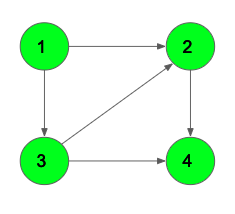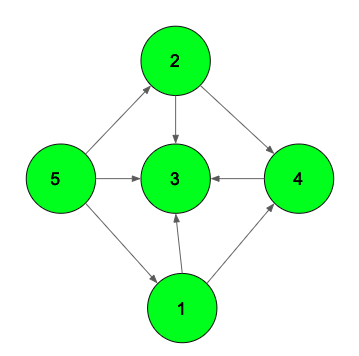# Longest path in a directed Acyclic graph | Dynamic Programming

Given a directed graph G with N vertices and M edges. The task is to find the length of the longest directed path in Graph.

Note: Length of a directed path is the number of edges in it.

Examples:

Input: N = 4, M = 5Output: 3
The directed path 1->3->2->4

Input: N = 5, M = 8Output: 3

## Recommended: Please try your approach on {IDE} first, before moving on to the solution.

Simple Approach: A naive approach is to calculate the length of the longest path from every node using DFS.

The time complexity of this approach is O(N2).

Efficient Approach: An efficient approach is to use Dynamic Programming and DFS together to find the longest path in the Graph.

Let dp[i] be the length of the longest path starting from the node i. Initially all positions of dp will be 0. We can call the DFS function from every node and traverse for all its children. The recursive formula will be:

dp[node] = max(dp[node], 1 + max(dp[child1], dp[child2], dp[child3]..))

At the end check for the maximum value in dp[] array, which will be the longest path in the DAG.

Below is the implementation of the above approach:

## C++

 `// C++ program to find the longest ` `// path in the DAG ` ` `  `#include ` `using` `namespace` `std; ` ` `  `// Function to traverse the DAG ` `// and apply Dynamic Programming ` `// to find the longest path ` `void` `dfs(``int` `node, vector<``int``> adj[], ``int` `dp[], ``bool` `vis[]) ` `{ ` `    ``// Mark as visited ` `    ``vis[node] = ``true``; ` ` `  `    ``// Traverse for all its children ` `    ``for` `(``int` `i = 0; i < adj[node].size(); i++) { ` ` `  `        ``// If not visited ` `        ``if` `(!vis[adj[node][i]]) ` `            ``dfs(adj[node][i], adj, dp, vis); ` ` `  `        ``// Store the max of the paths ` `        ``dp[node] = max(dp[node], 1 + dp[adj[node][i]]); ` `    ``} ` `} ` ` `  `// Function to add an edge ` `void` `addEdge(vector<``int``> adj[], ``int` `u, ``int` `v) ` `{ ` `    ``adj[u].push_back(v); ` `} ` ` `  `// Function that returns the longest path ` `int` `findLongestPath(vector<``int``> adj[], ``int` `n) ` `{ ` `    ``// Dp array ` `    ``int` `dp[n + 1]; ` `    ``memset``(dp, 0, ``sizeof` `dp); ` ` `  `    ``// Visited array to know if the node ` `    ``// has been visited previously or not ` `    ``bool` `vis[n + 1]; ` `    ``memset``(vis, ``false``, ``sizeof` `vis); ` ` `  `    ``// Call DFS for every unvisited vertex ` `    ``for` `(``int` `i = 1; i <= n; i++) { ` `        ``if` `(!vis[i]) ` `            ``dfs(i, adj, dp, vis); ` `    ``} ` ` `  `    ``int` `ans = 0; ` ` `  `    ``// Traverse and find the maximum of all dp[i] ` `    ``for` `(``int` `i = 1; i <= n; i++) { ` `        ``ans = max(ans, dp[i]); ` `    ``} ` `    ``return` `ans; ` `} ` ` `  `// Driver Code ` `int` `main() ` `{ ` `    ``int` `n = 5; ` `    ``vector<``int``> adj[n + 1]; ` ` `  `    ``// Example-1 ` `    ``addEdge(adj, 1, 2); ` `    ``addEdge(adj, 1, 3); ` `    ``addEdge(adj, 3, 2); ` `    ``addEdge(adj, 2, 4); ` `    ``addEdge(adj, 3, 4); ` ` `  `    ``cout << findLongestPath(adj, n); ` `    ``return` `0; ` `} `

## Java

 `// Java program to find the longest ` `// path in the DAG ` ` `  `import` `java.util.ArrayList; ` ` `  `// graph class ` `class` `Graph ` `{ ` ` `  `    ``int` `vertices; ` `    ``ArrayList edge[]; ` ` `  `    ``Graph(``int` `vertices)  ` `    ``{ ` `        ``this``.vertices = vertices; ` `        ``edge = ``new` `ArrayList[vertices+``1``]; ` `        ``for` `(``int` `i = ``0``; i <= vertices; i++)  ` `        ``{ ` `            ``edge[i] = ``new` `ArrayList<>(); ` `        ``} ` `    ``} ` `    ``void` `addEdge(``int` `a,``int` `b) ` `    ``{ ` `        ``edge[a].add(b); ` `    ``} ` ` `  `    ``void` `dfs(``int` `node, ArrayList adj[], ``int` `dp[], ` `                                    ``boolean` `visited[]) ` `    ``{ ` `        ``// Mark as visited ` `        ``visited[node] = ``true``; ` ` `  `        ``// Traverse for all its children ` `        ``for` `(``int` `i = ``0``; i < adj[node].size(); i++)  ` `        ``{ ` ` `  `            ``// If not visited ` `            ``if` `(!visited[adj[node].get(i)]) ` `                ``dfs(adj[node].get(i), adj, dp, visited); ` ` `  `            ``// Store the max of the paths ` `            ``dp[node] = Math.max(dp[node], ``1` `+ dp[adj[node].get(i)]); ` `        ``} ` `    ``} ` `     `  `    ``// Function that returns the longest path ` `    ``int` `findLongestPath( ``int` `n) ` `    ``{ ` `        ``ArrayList adj[] = edge; ` `        ``// Dp array ` `        ``int``[] dp = ``new` `int``[n+``1``]; ` ` `  `        ``// Visited array to know if the node ` `        ``// has been visited previously or not ` `        ``boolean``[] visited = ``new` `boolean``[n + ``1``]; ` ` `  `        ``// Call DFS for every unvisited vertex ` `        ``for` `(``int` `i = ``1``; i <= n; i++)  ` `        ``{ ` `            ``if` `(!visited[i]) ` `                ``dfs(i, adj, dp, visited); ` `        ``} ` ` `  `        ``int` `ans = ``0``; ` ` `  `        ``// Traverse and find the maximum of all dp[i] ` `        ``for` `(``int` `i = ``1``; i <= n; i++)  ` `        ``{ ` `            ``ans = Math.max(ans, dp[i]); ` `        ``} ` `        ``return` `ans; ` `    ``} ` `} ` ` `  `public` `class` `Main ` `{ ` `    ``// Driver code ` `    ``public` `static` `void` `main(String[] args) ` `    ``{ ` `        ``int` `n = ``5``; ` `        ``Graph graph = ``new` `Graph(n); ` `        ``// Example-1 ` `        ``graph.addEdge( ``1``, ``2``); ` `        ``graph.addEdge( ``1``, ``3``); ` `        ``graph.addEdge( ``3``, ``2``); ` `        ``graph.addEdge( ``2``, ``4``); ` `        ``graph.addEdge( ``3``, ``4``); ` `        ``graph.findLongestPath(n); ` `        ``System.out.println( graph.findLongestPath( n)); ` ` `  `    ``} ` `} ` ` `  `// This code is contributed by SumanSaurav `

## Python3

 `# Python3 program to find the  ` `# longest path in the DAG  ` `   `  `# Function to traverse the DAG  ` `# and apply Dynamic Programming  ` `# to find the longest path  ` `def` `dfs(node, adj, dp, vis):  ` `  `  `    ``# Mark as visited  ` `    ``vis[node] ``=` `True`  `   `  `    ``# Traverse for all its children  ` `    ``for` `i ``in` `range``(``0``, ``len``(adj[node])):   ` `   `  `        ``# If not visited  ` `        ``if` `not` `vis[adj[node][i]]: ` `            ``dfs(adj[node][i], adj, dp, vis)  ` `   `  `        ``# Store the max of the paths  ` `        ``dp[node] ``=` `max``(dp[node], ``1` `+` `dp[adj[node][i]])  ` `   `  `# Function to add an edge  ` `def` `addEdge(adj, u, v):  ` `  `  `    ``adj[u].append(v)  ` `   `  `# Function that returns the longest path  ` `def` `findLongestPath(adj, n):  ` `  `  `    ``# Dp array  ` `    ``dp ``=` `[``0``] ``*` `(n ``+` `1``)  ` `     `  `    ``# Visited array to know if the node  ` `    ``# has been visited previously or not  ` `    ``vis ``=` `[``False``] ``*` `(n ``+` `1``) ` `     `  `    ``# Call DFS for every unvisited vertex  ` `    ``for` `i ``in` `range``(``1``, n ``+` `1``):   ` `        ``if` `not` `vis[i]:  ` `            ``dfs(i, adj, dp, vis)  ` `      `  `    ``ans ``=` `0`  `   `  `    ``# Traverse and find the maximum of all dp[i]  ` `    ``for` `i ``in` `range``(``1``, n ``+` `1``):   ` `        ``ans ``=` `max``(ans, dp[i])  ` `      `  `    ``return` `ans  ` `  `  `# Driver Code  ` `if` `__name__ ``=``=` `"__main__"``:  ` `  `  `    ``n ``=` `5`  `    ``adj ``=` `[[] ``for` `i ``in` `range``(n ``+` `1``)] ` `   `  `    ``# Example-1  ` `    ``addEdge(adj, ``1``, ``2``)  ` `    ``addEdge(adj, ``1``, ``3``)  ` `    ``addEdge(adj, ``3``, ``2``)  ` `    ``addEdge(adj, ``2``, ``4``)  ` `    ``addEdge(adj, ``3``, ``4``)  ` `   `  `    ``print``(findLongestPath(adj, n)) ` `   `  `# This code is contributed by Rituraj Jain `

## C#

 `// C# program to find the longest ` `// path in the DAG ` `using` `System; ` `using` `System.Collections.Generic; ` ` `  `// graph class ` `class` `Graph ` `{ ` `    ``public` `int` `vertices; ` `    ``public` `List<``int``> []edge; ` ` `  `    ``public` `Graph(``int` `vertices)  ` `    ``{ ` `        ``this``.vertices = vertices; ` `        ``edge = ``new` `List<``int``>[vertices + 1]; ` `        ``for` `(``int` `i = 0; i <= vertices; i++)  ` `        ``{ ` `            ``edge[i] = ``new` `List<``int``>(); ` `        ``} ` `    ``} ` `    ``public` `void` `addEdge(``int` `a, ``int` `b) ` `    ``{ ` `        ``edge[a].Add(b); ` `    ``} ` ` `  `    ``public` `void` `dfs(``int` `node, List<``int``> []adj,  ` `                    ``int` `[]dp, Boolean []visited) ` `    ``{ ` `        ``// Mark as visited ` `        ``visited[node] = ``true``; ` ` `  `        ``// Traverse for all its children ` `        ``for` `(``int` `i = 0; i < adj[node].Count; i++)  ` `        ``{ ` ` `  `            ``// If not visited ` `            ``if` `(!visited[adj[node][i]]) ` `                ``dfs(adj[node][i], adj, dp, visited); ` ` `  `            ``// Store the max of the paths ` `            ``dp[node] = Math.Max(dp[node], 1 + ` `                                ``dp[adj[node][i]]); ` `        ``} ` `    ``} ` `     `  `    ``// Function that returns the longest path ` `    ``public` `int` `findLongestPath( ``int` `n) ` `    ``{ ` `        ``List<``int``> []adj = edge; ` `        ``// Dp array ` `        ``int``[] dp = ``new` `int``[n + 1]; ` ` `  `        ``// Visited array to know if the node ` `        ``// has been visited previously or not ` `        ``Boolean[] visited = ``new` `Boolean[n + 1]; ` ` `  `        ``// Call DFS for every unvisited vertex ` `        ``for` `(``int` `i = 1; i <= n; i++)  ` `        ``{ ` `            ``if` `(!visited[i]) ` `                ``dfs(i, adj, dp, visited); ` `        ``} ` ` `  `        ``int` `ans = 0; ` ` `  `        ``// Traverse and find the maximum of all dp[i] ` `        ``for` `(``int` `i = 1; i <= n; i++)  ` `        ``{ ` `            ``ans = Math.Max(ans, dp[i]); ` `        ``} ` `        ``return` `ans; ` `    ``} ` `} ` ` `  `class` `GFG ` `{ ` `    ``// Driver code ` `    ``public` `static` `void` `Main(String[] args) ` `    ``{ ` `        ``int` `n = 5; ` `        ``Graph graph = ``new` `Graph(n); ` `        ``// Example-1 ` `        ``graph.addEdge( 1, 2); ` `        ``graph.addEdge( 1, 3); ` `        ``graph.addEdge( 3, 2); ` `        ``graph.addEdge( 2, 4); ` `        ``graph.addEdge( 3, 4); ` `        ``graph.findLongestPath(n); ` `        ``Console.WriteLine(graph.findLongestPath(n)); ` `    ``} ` `} ` ` `  `// This code is contributed by Princi Singh `

Output:

```3
```

Time Complexity: O(N)
Auxiliary Space: O(N)

My Personal Notes arrow_drop_upCheck out this Author's contributed articles.

If you like GeeksforGeeks and would like to contribute, you can also write an article using contribute.geeksforgeeks.org or mail your article to contribute@geeksforgeeks.org. See your article appearing on the GeeksforGeeks main page and help other Geeks.

Please Improve this article if you find anything incorrect by clicking on the "Improve Article" button below.